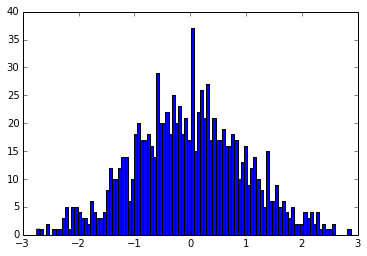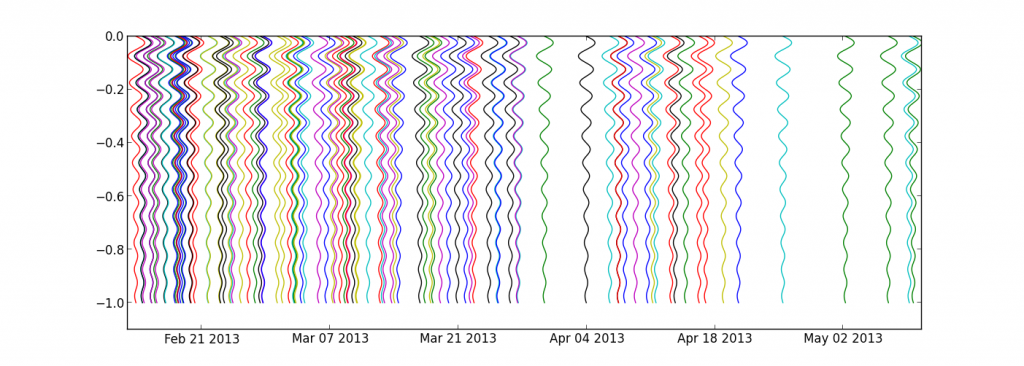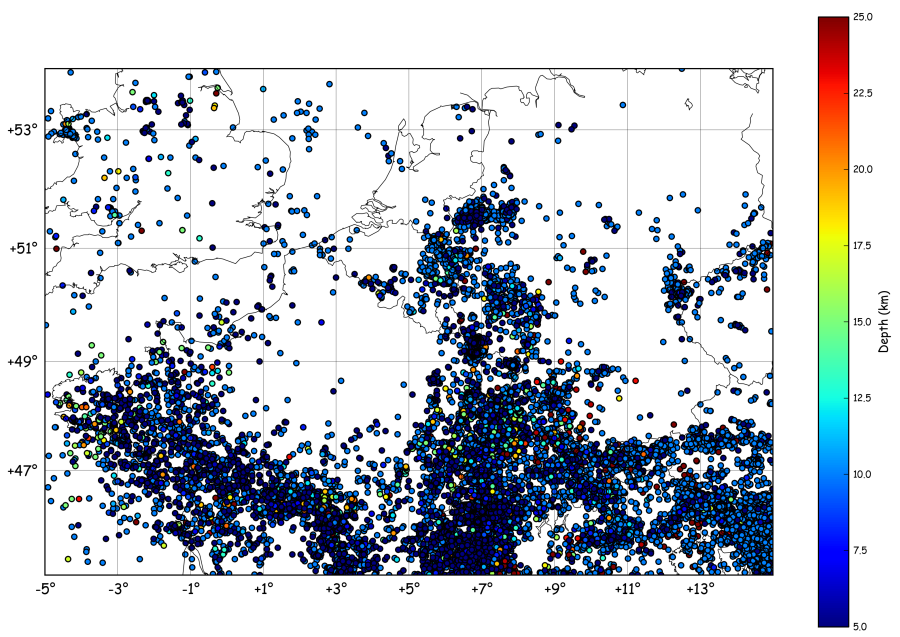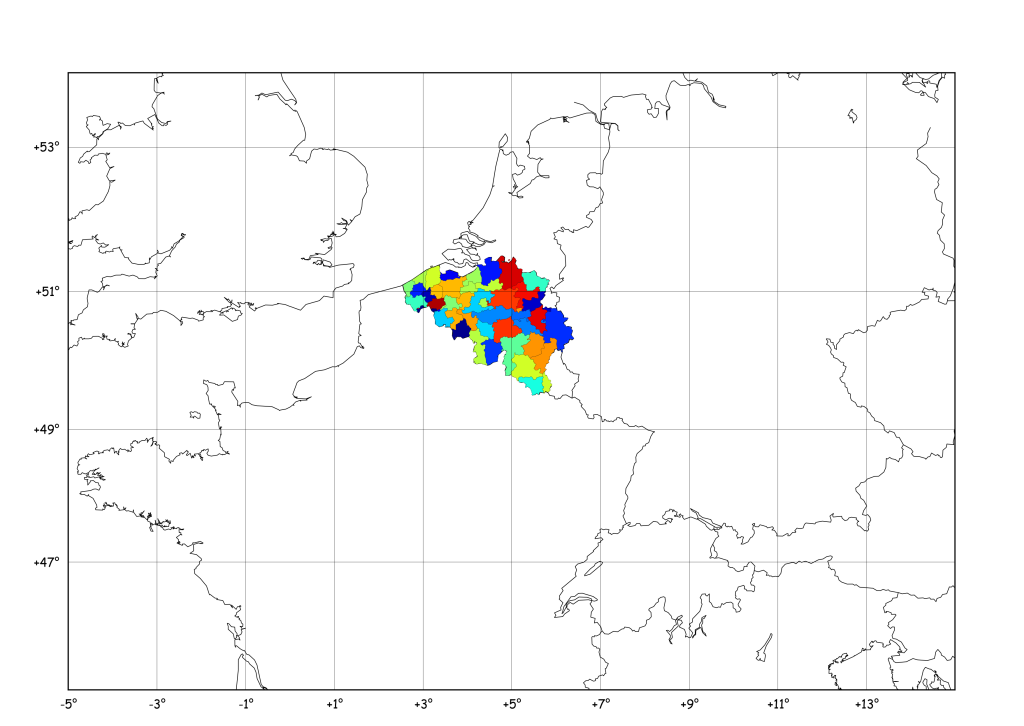## Jacknife

The Jacknife  is also sometimes called the “Leave One Out” method, and is a method to somehow evaluate the stability of statistics done on data. By leaving one element out of the input array and studying the mean of the…## Plot waveforms of events on a dates axis

Following a question from my dear colleague Devy, here is how to plot a set of events, occurring at random moments in time. The idea is to plot the waveform of each event with the beginning at the top and…## Seismicity Map and Rate using Basemap and Pandas

Imagine we want to plot a map of the seismic activity in NW-Europe and, at the same time, count the number events per month. To get a catalogue of earthquakes in the region, one can call the NEIC (note: this…## Matplotlib Basemap tutorial 10: Shapefiles Unleached, continued

Following the comments on this year-old post I’ve had a look at pyshp which seems a little more maintained (the last line in the changelog is 1 year old…), and it is indeed a quite nice piece of code. I…

## Matplotlib & Datetimes – Tutorial 04: Grouping & Analysing Sparse Data

To extend the previous tutorial (see here), we define a data array that has some information about the event that occurred for each datetime. The plot of data vs time now looks like: The data array is constructed with numpy.random:…

## Matplotlib & Datetimes – Tutorial 03: Grouping Sparse Data

New tutorial, more advanced this time ! Let’s say we have a number of observations, like occurrences of earthquakes, or visitors connecting to a webserver, etc. These observations don’t occur every second, they are sparse on the time axis. To…

## New on Géophysique.be: Gallery

Everyone used to search for code snippets on the web know the importance of a “Gallery” linking to all examples and tutorials… So, here is my really ugly Gallery :

## Matplotlib Basemap tutorial 08: Shooting Great Circles

Following a question from Ricardo Gama (see his comment), I’ve prepared this new tutorial. He wondered if Basemap has a function similar to the track1 function in matlab (you know, that crappy costly thing…)… Here is what I obtained :…

## Matplotlib Basemap tutorial 05 : Adding some pie charts !

Hi folkes, Following a question by Gui on this website, I checked the “how to” draw pie charts on a basemap plot ! Here below I generalise the example given by Manuel Metz on the matplotlib website. The idea is…

## Matplotlib Basemap tutorial 03 : Masked arrays & Zoom

Here, we will focus on adding a “zoom box” on the top left corner of the plot. But before that, we will mask a part of the earthquakes, in order to have a “cleaner” map ! This is achieved by…

## Matplotlib Basemap tutorial 02 : Let’s add some earthquakes !

Now, let’s imagine we have a dataset containing latitude/longitudes of earthquakes, plus their depth and magnitude. Of course, you don’t always have this dataset available, so let’s build a fake one : import numpy as np lon = np.random.random_integers(11,79,1000)/10. lat…

## Matplotlib Basemap tutorial 01 : Your first map

# # BaseMap example by geophysique.be # tutorial 01 from mpl_toolkits.basemap import Basemap import matplotlib.pyplot as plt import numpy as np fig = plt.figure(figsize=(11.7,8.3)) #Custom adjust of the subplots plt.subplots_adjust(left=0.05,right=0.95,top=0.90,bottom=0.05,wspace=0.15,hspace=0.05) ax = plt.subplot(111) #Let’s create a basemap around Belgium m…